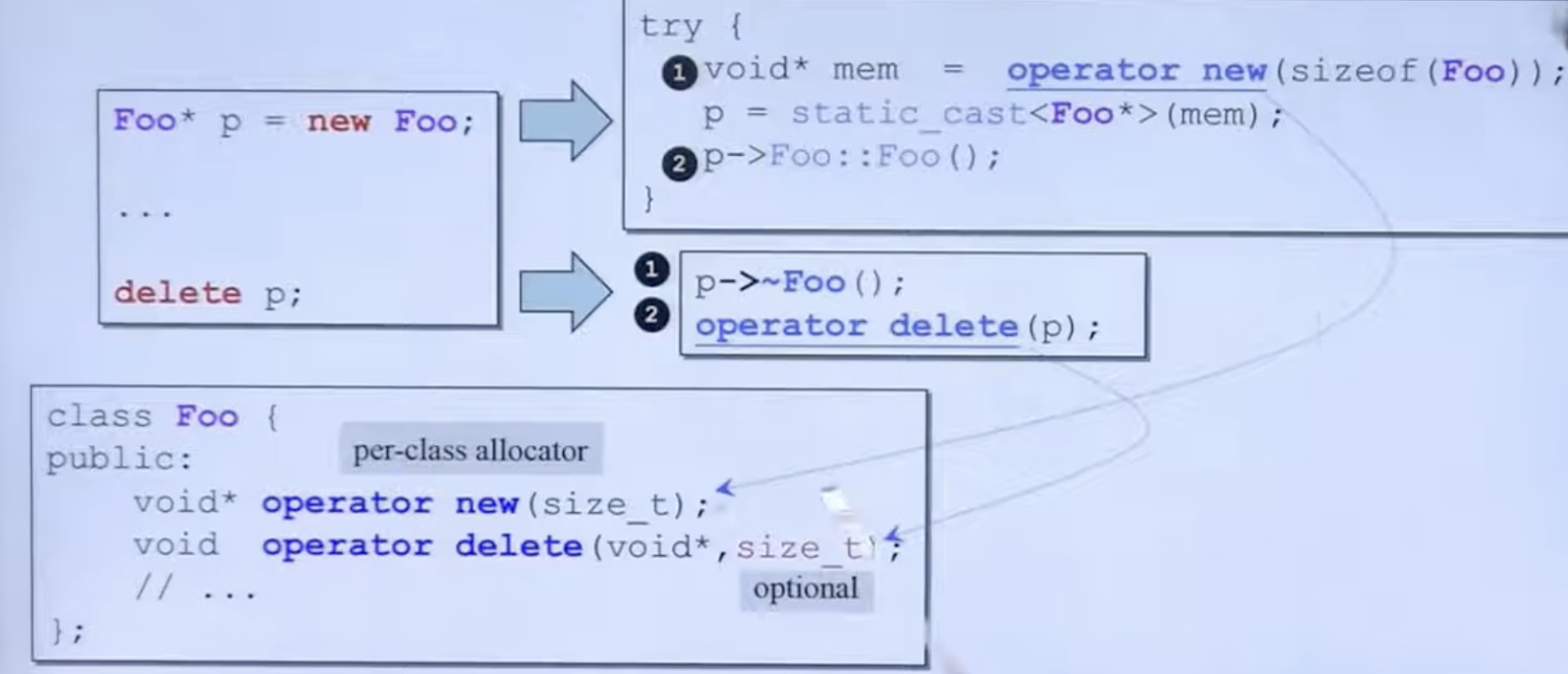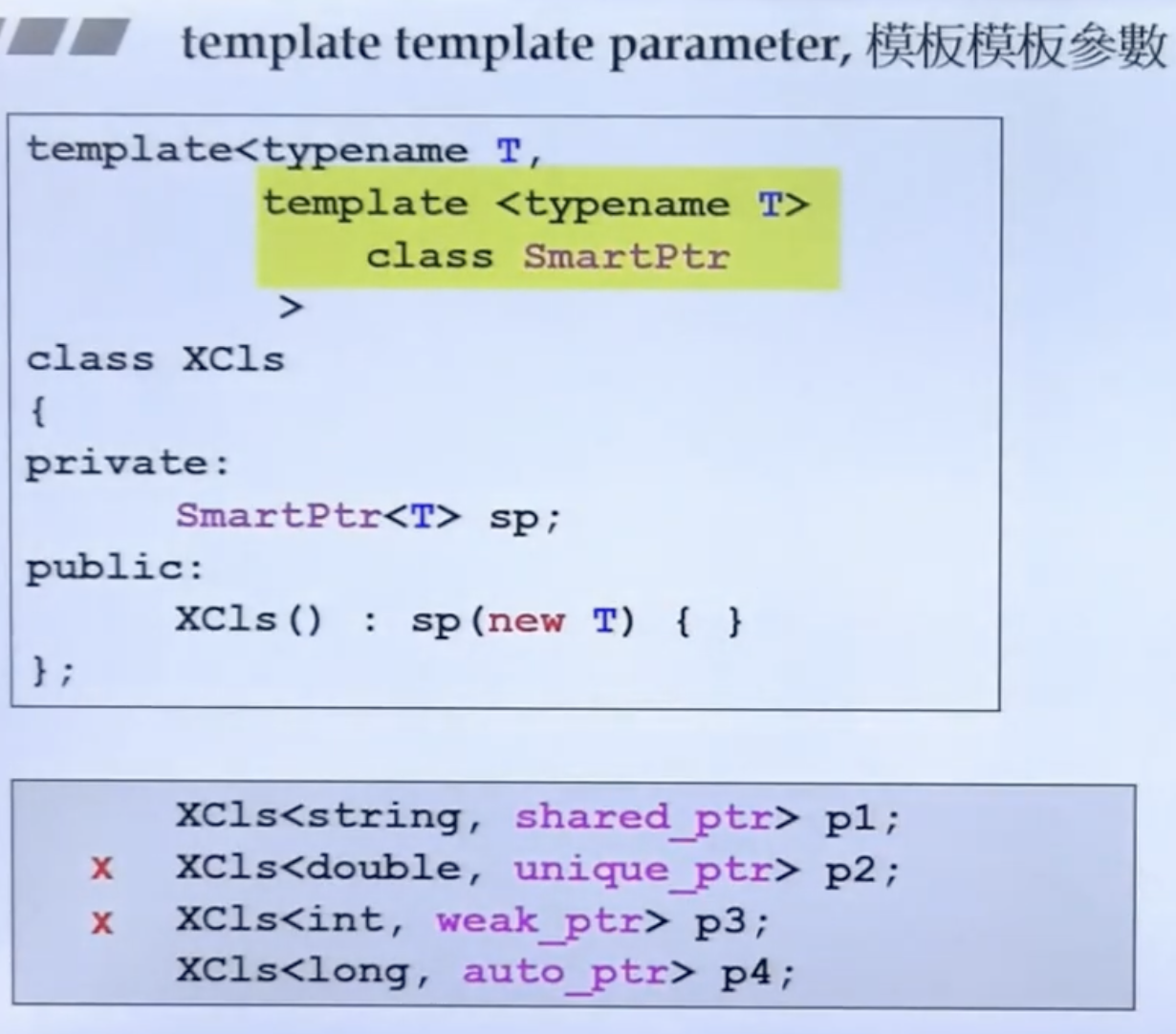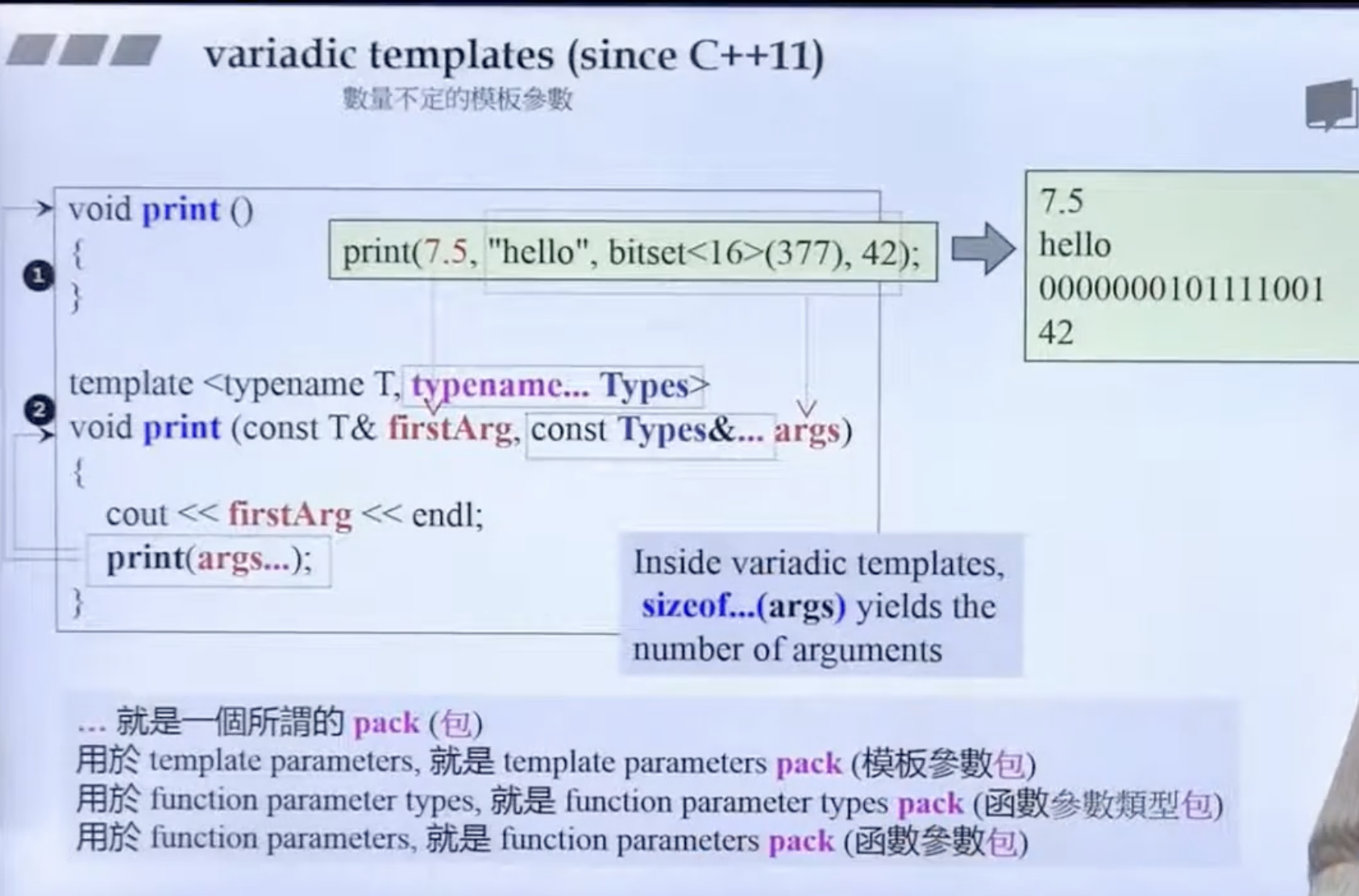yzzheng

V1

2023/04/11阅读：9主题：红绯

# houjie 面向对象

## 面向对象（上）

### const

const还是属于函数签名的一部分。 引用计数：涉及到共享的东东，然后当某个修改的时候，使用COW（Copy on Write）

### new 和 delete

• 重载member operator new/delete• 重载成员操作符 new[] / delete[]

## 面向对象（下）

### (3) non-explicit-one-argument ctor

``#include<iostream>using namespace std;class Fraction{public:    Fraction(int num, int den = 1):m_numerator(num), m_denominator(den){    }    Fraction operator+(const Fraction& fra){        //todo 需要修改规则        return Fraction(this->m_numerator+fra.m_numerator,        this->m_denominator + fra.m_denominator);    }    // operator double() const{    //     //  error: use of overloaded operator '+' is ambiguous (with operand types 'Fraction' and 'int')    //      因为这里存在两条分支路可以编译代码    //     return (double)(m_numerator / m_denominator);    // }    int get_m_numberator() const {        return this->m_numerator;    }       int get_m_denominator() const{        return this->m_denominator;    }private:    int m_numerator;    int m_denominator;};inline std::ostream& operator<< (std::ostream& os, const Fraction& o){    return os << "(" << o.get_m_numberator() << ", " << o.get_m_denominator() << ")" << endl;}int main(){    Fraction f(3,5);    Fraction d2 = f + 4;    // 这里会把4转换为Fraction(4, 1)    cout << d2 << endl;     return 0;}``

explitcit-one-argument ctor 给Fraction 加上explicit，会要求编译器不用把4强制转换为Fraction

conversion function 转换函数 没有返回类型，返回类型就是operator double()

### （4）pointer-like classes 智能指针

``#include<iostream>using namespace std;template<class T>class my_shared_ptr{public:    T& operator*() const{        return *px;    }    T* operator->() const{        return px;    }    my_shared_ptr(T* p):px(p){    }private:    T* px;    long* pn;};struct Foo{  void method(void){    cout << "call Foo.method" << endl;  }  };int main(){    my_shared_ptr<Foo> sp(new Foo);    Foo f(*sp);    sp->method();   // -> 函数返回一个px指针，但是->可以继续使用所以等价于 px->method()    return 0;}``

### 12 模板模板参数

shared_prt 和 auto_ptr### 14 三个主题 C++ 11

1. 数量不定的模板参数1. auto

2. range-base for

### 15 引用

int* ： pointer 2 integer int& ： reference 2 interger

reference 一定要有初值，指针可以变化，reference 不可以变化

### 16 复合 & 继承

1. inheritance 继承关系下的构造和析构

1. composition 复合关系下的构造和析构
1. Inheritance + composition关系下的析构和构造

### 19 关于动态绑定V1

hello